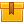# Multimeters (A8002)

## Prerequisites

This lesson is designed so that no prior knowledge is required.

## Description

This lesson demonstrates and explains how to use both a digital and an analog multimeter. During this lesson, voltage, resistance, current, capacitance, and frequency are measured. This lesson also describes some of the more common features of a digital multimeter.

## Objectives

Identify and describe the display area, the function switch, and the leads/jacks on a digital multimeter. Adjust the mechanical zero and interpret a reading on the voltage scale, and the resistance scale of an analog meter. Given an expected measurement, set the function and range switches of an analog multimeter and adjust the zero on the ohms scale. Know how to use a multimeter for a variety of purposes including checking for continuity across a circuit, measuring resistance, current, frequency, ac and dc voltage, and capacitance. And use the hold button, relative button, range button, and min/max button on a DMM.

## ContentThis lesson demonstrates and explains how to use both a digital and an
analog multimeter. During this lesson, voltage, resistance, current,
capacitance, and frequency are measured. This lesson also describes some
of the more common features of a digital multimeter.

•Multimeters (A8002)

0
0 Reviews